### Home > CALC > Chapter 4 > Lesson 4.2.3 > Problem4-80

4-80.
1.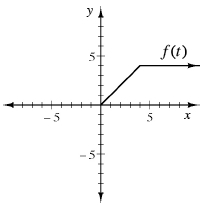Use the partial graph of f(t) shown at right to complete the following questions. 4-80 HW eTool (Desmos). Homework Help ✎

1. If f(t) is an odd function and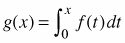, evaluate:

1. g(−3)

2. 2 · g(−7)

3. g(9) + g(−9)

2. If f(t) is an even function and, evaluate:

1. g(−3)

2. 2 · g(−7)

3. g(9) + g(−9)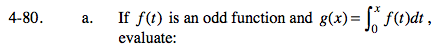Sketch the graph of the even function, f(t).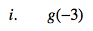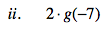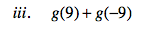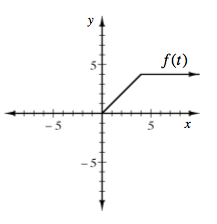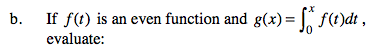Sketch the graph of the odd function, f(t).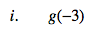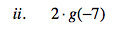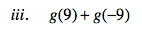Use the eTool below to help solve this problem.
Click the link at right for the full version of the eTool: Calc 4-80 HW eTool